Binary Numbering System

The following 2 sections on binary numbering system and memory are optional but recommended. If you’ve taken a course in electronics you probably already know about this and can use the material provided here as a refresher. Having knowledge of the binary system and computer memory will help in understanding some features in programming.

The heart of the computer is the microprocessor, which is also referred to as the processor. The microprocessor is the main part of the computer’s CPU (central processing unit). A processor consists of millions of electronic switches; a switch can be either in ON or OFF state. Thus there are only two distinct states possible in these devices. In our real-world calculations we make use of the decimal system (which has 10 distinct states/numbers: 0 to 9). Counting up to ten is easy for humans but would be quite difficult for computers. It is easier to create a device capable of sensing 2 states than one capable of sensing 10 states (this also helps reduce on errors). Computers make use of the binary system (i.e. they store data in binary format and also perform calculations in binary format). The binary system (binary meaning two) has just two numbers: 1 and 0 (which correspond to the ON and OFF state respectively – this is analogous to a switch which can either be in ON state or in OFF state).

When we have different systems (binary, decimal etc.), there ought to be a way of converting data from one system to the other. In the decimal system the number 247 stands for 7*100 + 4*101 + 2*102 (add it up and the result will be 247; i.e. in each place we can have one of the 10 digits and to find the actual place value we have to multiply the digit by the corresponding power of 10). For example:

247 = (2 x 102) + (4 x 101) + (7 x 100) = 200 + 40 + 7

1258 = (1 x 103) + (2 x 102) + (5 x 101) + (8 x 100) = 1000 + 200 + 50 + 8

Note: In C++ and most other computer languages, * is used as the multiplication operator.

The same concept holds good for a binary number but since only two states are possible, they should be multiplied by powers of 2.

Remember: A binary digit is called a bit.

So, what is the value of 1101? Multiply each position by its corresponding power of 2 (but remember, you have to start from 20 and not from 21). The value for 1101 is 13 as illustrated in the figure below: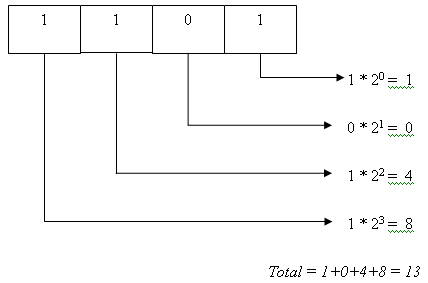An alternate method to obtain the value is illustrated below (but the underlying concept is the same as above):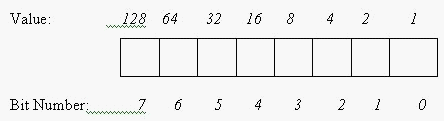It is easy to obtain the values which are written above each bit (27=128, 26=64 and so on). Write these values on top and then write the binary number within the squares. To find the equivalent decimal value, add up the values above the square (if the number in the square is 1). If a number is denoted as 1101, then this stands for the lower (or last) four bits of the binary number (the upper bits are set to 0). Hence 1101 will come under the values 8, 4, 2 and 1. Now, wherever there is a 1, just add the value above it (8+4+1=13). Thus 13 is the decimal equivalent of 1101 (in binary format). To distinguish between decimal and binary we usually represent the system used (decimal or binary) by subscripting the base of the system (10 is the base for the decimal system while 2 is the base for the binary system).

Hence (13)10 = (1101)2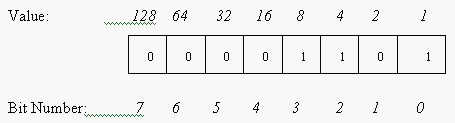Computers store information in the form of bits and 8 bits make a byte. But memory capacity is expressed as multiples of 210 bytes (which is equal to 1024 bytes). 1024 bytes is called a Kilobyte. You may wonder why it is 1024 and not 1000 bytes. The answer lies in the binary system. Keeping uniformity with the binary system, 210=1024 and not 1000 (the idea is to maintain conformity with the binary system).

Beware: The bit in position 7 in fig 1.1 is actually the 8th bit of the number (the numbering of bit starts from 0 and not 1). The bit in the highest position is called as the most significant bit. In an 8 bit number, the 7th bit position is called the most significant bit (MSB) and the 0th bit is known as the least significant bit (or LSB). This is because the MSB in this case has a value of 128 (28) while the LSB has a value of just 1.

Computer Memory

We know that computers can operate only on bits (0s and 1s). Thus any data that has to be processed by the computer should be converted into 0s and 1s.

Let us suppose that we want to create a text file containing a single word “Hello”. This file has to be stored physically in the computer’s memory so that we can read the file anytime in the future. For the time being forget about the file-storage part. Let’s just concentrate on how the word “hello” is stored in the computer’s memory. Computers can only store binary information; so how will the computer know which number corresponds to which alphabet? Obviously we cannot map a single bit to a character. So instead of bits we’ll consider a byte (8 bits). Now we can represent 256 characters. To perform map a character to a byte we’ll need to use some coding mechanism. For this purpose the ASCII (American Standard Code for Information Interchange) is used. In this coding system, every alphabet has an equivalent decimal value. When the computer uses ASCII, it cannot directly use the decimal value and it will convert this into an 8-bit binary number (in other words, into a byte) and store it in memory.

The following table shows part of the ASCII code.

 Character Equivalent decimal value Binary value A 65 0100 0001 B 66 0100 0010 a 97 0110 0001 b 98 0110 0010

In this way each character is mapped to a numeric value. If we type the word hello, then it is converted into bytes (5 bytes- one for each character) based on the ASCII chart and is stored in memory. So ‘hello’ occupies 5 bytes or 40 bits in memory.

Note: It is very important to know the binary system if you want to use the bitwise operators available in C++. The concept of memory is useful while learning pointers.

A question arises, “where are the individual bits stored in memory?” Each individual bit is stored in an electronic device (the electronic device is technically called a flip-flop; which is something like a switch). A single flip-flop can store one bit. Consider the fig. below: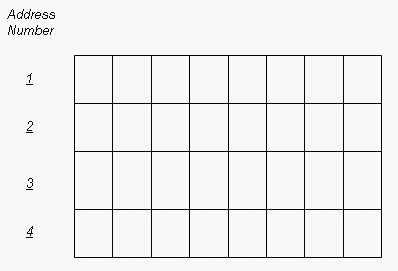As mentioned earlier we deal in terms of bytes rather than bits. The figure shows a 4-byte memory (which means it can hold 32 bits – each cell can store one bit). All information is stored in memory and the computer needs some method to access this data (i.e. there should be some way of distinguishing between the different memory locations). Memory addresses serve this purpose. Each bit can be individually accessed and has a unique memory address. To access the first byte, the computer will attempt to read the byte stored at memory address 1.

If memories didn’t have addresses then the computer would not know from where it has to read data (or where it has to store data). An analogy to memory address is the postal address system used in real-life. A city will have a number of houses and each house has a unique address. Just imagine the situation if we didn’t have any postal address (we wouldn’t be able to locate any house in the city!). One difference is that a memory address can house only bits and nothing else.

Memory address representations are not as simple as shown above. In the above case we’ve considered a memory that has capacity to store just 4 bytes. Memories usually contain kilobytes or gigabytes of space. As the amount of memory available increases, so does the size of the address (the address number might be in the range of millions). Thus instead of using the decimal system for addresses, the hexadecimal numbering system is used. Hexa means 16 and the distinct numbers in this system are 0 to 9 followed by A, B, C, D, E and F where A= 10 in decimal, B= 11 in decimal and F= 15 in decimal.

Counting in hexadecimal system will be 0…9, A, B…F, 10,11,12,13…19,1A, 1B, 1C…and so on.

It is quite clear that 10 in hexadecimal does not equal 10 in decimal. Instead, (0F)16 = (15)10, (10)16 = (16)10 and (11)16 = (17)10

Four bits form a ‘nibble’. Eight bits form a ‘byte’ and four bytes of memory are known as a ‘word’. There is some significance attached to a ‘word’ which we shall deal with later.

Another term related to memory is ‘register’. A register consists of a set of flip-flops (flip-flops are electronic devices that can store one bit) for storing information. An 8-bit register can store 8 bits. Every processor has a set of internal registers (i.e. these registers are present within the processor and not in an external device like a hard disk). These registers (which are limited in number depending on the processor) are used by the processor for performing its calculations and computations. They usually contain the data which the processor is currently using. The size of the register (i.e. whether the registers will be 16-bit, 32-bit or 64-bit registers also depends on the processor). The common computers today use 32-bit registers (or 4-byte registers). The size of a register determines the ‘word-size’ of a computer. A computer is more comfortable (and prefers) working with data that is of the word-size (if the word-size is 4 bytes then the computer will be efficient in computations involving blocks of 4 byte data). You’ll understand this when we get into data types in the subsequent chapters.

The different types of memory are:

1.      Secondary storage (for example the hard disk, floppy disks, magnetic disks, CD-ROM) where one can store information for long periods of time (i.e. data is retained in memory irrespective of whether the system is running or not).

2.      The RAM (random access memory) is used by the computer to store data needed by programs that are currently running. RAM is used for the main (primary) memory of the computer (all programs which are executed need to be present in the main memory). The RAM will lose whatever is stored in memory once the computer is switched off.

3.      ROM (read only memory): This contains instructions for booting up the system and performing other start-up operations. We cannot write to this memory.

4.      The internal registers within the processor- these are used by the computer for performing its internal operations. The compiler will decide what has to be stored in which register when it converts our high-level code into low-level language. As such we won’t be able to use these registers in our C++ code (unless we write assembly code).

Remember: Secondary storage (also called auxiliary memory) is not directly accessible by the CPU. But RAM is directly accessible (thus secondary memory is much slower than the primary memory). For a program to execute it needs to be present in the main memory.

Which memory has lowest access time (or which memory can the CPU access quickly)?

The internal registers can be accessed quickly and the secondary storage devices take much longer to access. Computers also make use of a cache-memory. Cache memory is a high-speed memory which stores recently accessed data (cache access is faster than main memory access).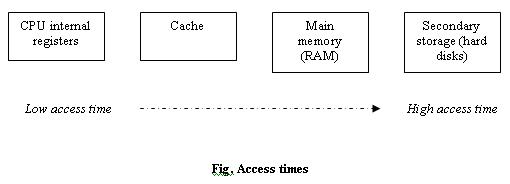Related concept: In general cache means storing frequently accessed data temporarily in a place where it can be accessed quickly. Web browsers tend to cache web pages you visit frequently on the hard disk. Generally when we type in a website address, the browser needs to query the website and request for the page; which is a time consuming process. When the browser displays this webpage, it internally caches (stores a copy) this webpage on our hard disk also. The next time we time the same website address, the browser will directly read out from the hard disk rather than query the website (reading from hard disk is faster than accessing a web server).

Remember: Computers store information using the binary system, addresses are represented in the hexadecimal system and in real-life we use the decimal system.

A 3-bit number can be used to form 8 different combinations (including the 000 combination).

 Binary Decimal Equivalent 000 0 001 1 010 2 011 3 100 4 101 5 110 6 111 7

If 3 bits are used then the maximum possible decimal number that can be represented is 7 (not 8 because the first number is 0). Similarly if an 8-bit number can be used to represent up to (2^8) different values (0 to 255 in the decimal system).

A binary number can be either signed or unsigned. When a number is unsigned (we don’t bother about the sign), it means that the number is always positive. If a number is signed, then it could be positive or negative. +33 is a signed number (with a positive sign). In real life, we can use + or – to indicate whether a number is positive or negative but in computers only 1s and 0s can be used. Every decimal number has a binary equivalent. The binary equivalent for the decimal number 8 is 00001000. If this is a signed number then +8 would be written as 00001000 and –8 would be denoted by 10001000. Notice the difference between the two. The 7th bit (or the most significant bit) is set to 1 to indicate that the number is negative.

Assume the number 127.

For +127 you will write: 01111111

For –127 you will write: 11111111

For 255 you will write: ?

Well, the value for 255 cannot be written using a signed 8-bit number (because the 7th bit is reserved for the sign). If the unsigned representation was used then 255 can be represented as 11111111 (in this case the MSB signifies a value and is not concerned about the sign of the number).

What is the point to note here? By using a signed representation the maximum value that can be represented is reduced. An 8 bit unsigned binary number can be used to represent values from 0 to 255 (11111111 will mean 255). On the other hand, if the 8 bit binary number is a signed number then it can represent from –127 to +127 only (again a total of 255 values but the maximum value that can be represented is only 127).

Beware: Signed representation in binary format will be explained in detail later. Computers store negative numbers in 2s complement rather than storing them directly as shown above.

Remember: In signed numbers the MSB (in the binary representation) is used to indicate the sign of the number.

Go back to the Contents Page